• 拉普拉斯变换Matlab求解 知识点K1.12 拉普拉斯变换Matlab求解 主要内容 拉普拉斯变换Matlab求解 基本要求 掌握拉普拉斯变换Matlab函数 1 Xidian University, ICIE. All Rights Reserved 拉普拉斯变换的...
• 拉普拉斯变换Matlab求解 知识点K1.12 拉普拉斯变换Matlab求解 主要内容 拉普拉斯变换Matlab求解 基本要求 掌握拉普拉斯变换Matlab函数 1 拉普拉斯变换Matlab求解 K1.12 拉普拉斯变换Matlab求解 MATLAB ...
• 示例 1 ODE 拉普拉斯变换特奥多西奥，克里斯蒂安·罗马一世。 MATH156/E02
• 精品文档 精品文档 PAGE PAGE #欢迎下载 实验六拉普拉斯变换及其逆变换 一 目的 掌握连续系统及信号拉普拉斯变换概念 掌握利用MATLAB^制系统零极点图的方法 掌握利用MATLAB^解拉普拉斯逆变换的方法 二 拉普拉斯变换...
• 我们新的数值拉普拉斯逆变换过程快速，简单，但仍比最常用的替代过程优越得多。 它没有过冲和下冲，因此非常适合反转概率分布的拉普拉斯变换，因为它永远不会为它们返回负值或一个以上的结果。
• 这是关于拉普拉斯变换matlab程序，我感觉挺有用 可以下来看看
• 交互式应用程序，展示了脉冲信号拉普拉斯变换的 3D 可视化及其与傅立叶变换的关系。 脉冲信号的宽度和切口在 s 平面上的轨迹可以改变。 可以从不同的视角查看拉普拉斯变换 X(s) 的幅度。 需要 R2014b 或更新版本。 ...
• % 示例 1 f1 = @(t) t.^2; Lapf1 = @(s) LapTrans(f1,s); sc = 1:0.1:10; sp = 1:10; yc = 2./sc.^3; yp = arrayfun(Lapf1, sp); 图1） 情节（sc，yc，'r'，sp，yp，'*'）；... title（'数字拉普拉斯变换（sin
• laplace(ft) 单边拉普拉斯变换 ilaplace(Fs) 拉普拉斯逆变换 num=[1 0];den=[1 0 100]; %X=s/(s^2+100) sys=tf(num,den); %建立一个传递函数，分子为num,分母为den poles=roots(den) %求极点 pzmap(sys); %零极点...
**
所用的MATLAB函数：
**

dirac(t) 冲激函数   heaviside(t)  阶跃函数
laplace(ft)  单边拉普拉斯变换    ilaplace(Fs) 拉普拉斯逆变换
num=[1 0];den=[1 0 100];  %X=s/(s^2+100)
sys=tf(num,den);  %建立一个传递函数，分子为num,分母为den
poles=roots(den)  %求极点
pzmap(sys);  %零极点分布图显示
[r,p,k]=residue(num,den)
num den分子分母多项式的系数向量
r部分分式的系数
p为极点
k为多项式系数，若为真分式，其为0
roots(den)函数计算H（s）的零极点
format rat   %使用分数来表示数值
pretty(Ys);%看起来好看的Ys
yzit=vpa(yzit0,4);  %vpa 设置精度，0.001111 四位
t1=linspace(eps,5,100); %eps = 1/4503599627370496=0+；
ht1=subs(ht,t,t1); %置换函数 syms t->t1数值点，求冲激函数各时点的数值解
t=0:0.02:15;mpulse(num,den,t);step(num,den,t); 冲激响应 阶跃响应绘图
[H,w]=freqs(num,den); plot(w,abs(H))；plot(w,angle(H))求得幅频相频响应

正文
**
**
1.从傅里叶变换到拉普拉斯变换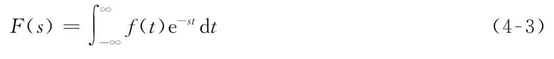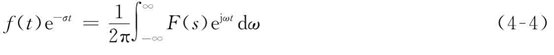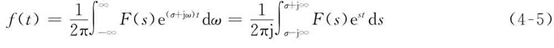2.双边拉普拉斯变换的收敛域（1）	因果的。当t＜0时，f（t）=0。若因果信号的双边拉普拉斯变换存在，则其收敛域在其极点右边的一个平面。
（2）	反因果的。当t＞0时，f（t）=0。若反因果信号的双边拉普拉斯变换存在，则其收敛域在其极点左边的一个平面。
（3）非因果的，以上二者的组合。若非因果信号的双边拉普拉斯变换在，则其收敛域是其因果部分的收敛域和其反因果部分的收敛域的交集。
3.单边拉普拉斯变换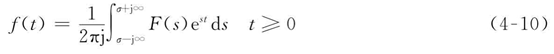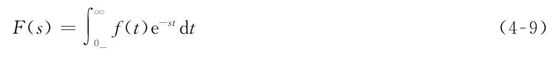利用MATLAB求解laplace求信号的单边拉普拉斯变换
符号法：
syms t s;
d=dirac(t);
D=laplace(d)
u=heaviside(t);
U=laplace(u)
g=heaviside(t+1)-heaviside(t-1);
G=laplace(g)
x=exp(-2*t);
X=laplace(x)

结果：
D =1
U = 1/s
G =1/s - exp(-s)/s
X =1/(s + 2)
3.单边拉普拉斯变换的性质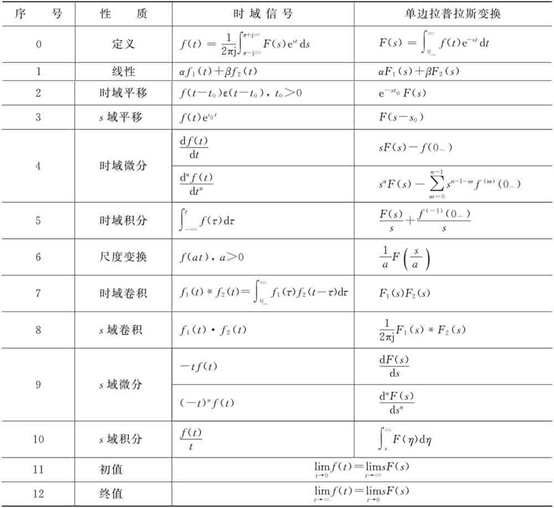MATLAB比较cos10t ε（t）和e–tcos10t ε（t）的极点位置，分析s域平移性质对收敛域的影响
符号计算法
clear
syms t s;
x=cos(10*t);
y=exp(-t)*cos(10*t);
X=laplace(x)   %好像laplace函数就是求单边普，不用x=cos(10*t)*heaviside(t)了
Y=laplace(y)    % plotting of signals and poles/zeros??
figure(1)
subplot(221)
ezplot(x,[0,5]);grid
axis([0 5 -1.1 1.1]);
title('x(t)=cos(10t)ε(t)')
num=[1 0];den=[1 0 100];  %X=s/(s^2+100)可知
sys=tf(num,den);  %建立一个传递函数，分子为num,分母为den
poles=roots(den)  %求极点
subplot(222)
pzmap(sys);  %零极点分布图显示
axis([-2 1 -20 20]);
subplot(223)
ezplot(y,[-1,5]);grid
axis([0 5 -1.1 1.1]);
title('y(t)=cos(10t)exp(-t)ε(t)')
num=[0 1 1];den=[1 2 101];
sys=tf(num,den);
poles=roots(den)
subplot(224)
pzmap(sys);
axis([-2 1 -20 20]);

X =
s/(s^2 + 100)
Y =
(s + 1)/((s + 1)^2 + 100)
poles =
0.0000 +10.0000i
0.0000 -10.0000i
poles =
-1.0000 +10.0000i
-1.0000 -10.0000i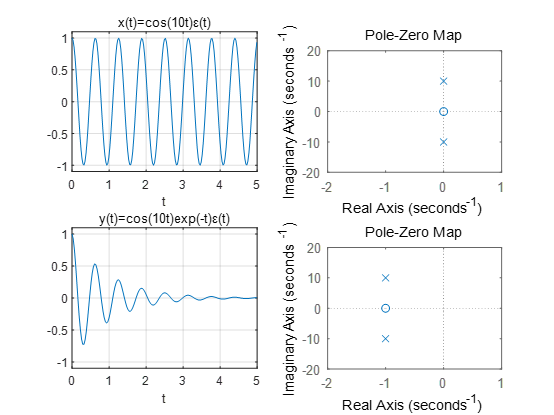4.单边拉普拉斯逆变换
4.1 部分分式展开法
1.单极点 实数 2.共轭复极点
format rat   %使用分数来表示数值
num=[3 11 15 6];
den=[1,3,2];
[r,p,k]=residue(num,den)
r =
4
-1
p =
-2
-1
k =
3              2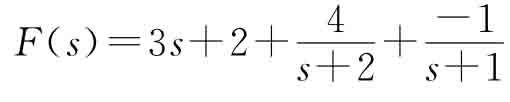%F（s）=s^2-4/(s^2+4s+8)(s+3)
num=[1 0 -4];
den=conv([1 4 8],[1 3]); %卷积求多项式乘
[r,p,k]=residue(num,den)
magr=abs(r)   %求r得模
angr=angle(r)  %求r得相∩

r=1+0i,1/321685687669322+1i,1/321685687669322-1i
p=-3+0i,-2+2i,-2-2i
magr=1,1,1
angr=0,355/226,-355/226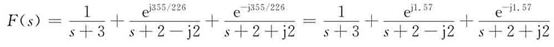5 连续系统的拉普拉斯分析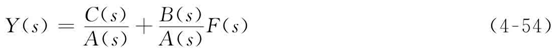完全响应=零输入响应+零状态响应
已知线性时不变系统的微分方程为
y″（t）+3y′（t）+2y（t）=f′（t）+3f（t）
输入信号f（t）=e–4tε（t），y（0–）=1，y′（0–）=1，求系统的零输入响应、零状态响应和全响应。
%y''+3y'+2y=f'+3f
syms t s;
ft=exp(-4*t)*heaviside(t); %输入信号
a=[1 3 2];b=[1 3];%微分方程系数向量
y0=[1 1];   %系统初始状态 y0(1)=y(0-) y0(2)=y'(0-)
Fs=laplace(ft);
%%计算Cs即C（s）
n=length(a)-1;Cs=0;
for k=1:n;
for r=0:(k-1);
Cs=Cs+a(n-k+1)*y0(r+1)*s^(k-1-r);
end
end
As=s^2+3*s+2;Bs=s+3;
Hs=Bs./As;
ht=ilaplace(Hs);disp('系统冲激响应');ht
Ys=Cs/As+Bs/As*Fs;disp('Y(s)=');
pretty(Ys);%看起来好看的Ys
yt=ilaplace(Ys);disp('全响应');yt
yzit0=ilaplace(Cs/As);yzit=vpa(yzit0,4);  %vpa 设置精度，0.001111 四位
disp('零输入响应');yzit
disp('零输入响应');yzit
yzst0=ilaplace(Bs/As*Fs);yzst=vpa(yzst0,4);
disp('零状态响应');yzst
%%绘图
t1=linspace(eps,5,100); %eps = 1/4503599627370496=0+；
ht1=subs(ht,t,t1); %置换函数 syms t->t1数值点，求冲激函数各时点的数值解
subplot(211);plot(t1,ht1);
title('冲激响应');grid;
yt1=subs(yt,t,t1);subplot(212);plot(t1,yt1,'r--');
yzit1=subs(yzit,t,t1);hold on;plot(t1,yzit1,'k--');
yzst1=subs(yzst,t,t1);hold on;plot(t1,yzst1,'b--');
title('系统响应')
legend('全响应','零输入','零状态');

系统冲激响应
ht =
2*exp(-t) - exp(-2*t)
Y(s)=
s + 4               s + 3
------------ + ----------------------
s ^2 + 3 s + 2   (s + 4) (s^2  + 3 s + 2)
全响应
yt =
(11*exp(-t))/3 - (5*exp(-2*t))/2 - exp(-4*t)/6
零输入响应
yzit =
3.0*exp(-1.0*t) - 2.0*exp(-2.0*t)
零状态响应
yzst =
0.6667*exp(-1.0*t) - 0.5*exp(-2.0*t) - 0.1667*exp(-4.0*t)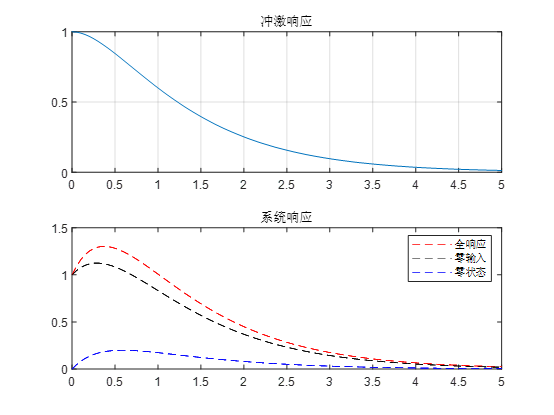已知线性时不变系统的系统函数H（s）=s+2/s^2+4
，输入信号f（t）=ε（t），求系统的零状态响应。

syms t s;
ft=heaviside(t);
Fs=laplace(ft);
As=s^2+4;Bs=s+2;Hs=Bs./As;
ht=ilaplace(Hs);
yzst0=ilaplace((Bs*Fs)/As);yzst=vpa(yzst0,4);
disp('零状态响应'),yzst,
t1=linspace(eps,5,100);
ht1=subs(ht,t,t1);
subplot(2,1,1);plot(t1,ht1),
xlabel('时间(秒)'),ylabel('幅度') ,grid,title('冲激响应'),
subplot(2,1,2);
yzst1=subs(yzst,t,t1);hold on;plot(t1,yzst1,'b-.')
xlabel('时间(秒)'),ylabel('幅度') ,grid,title('零状态响应')


6.系统函数与系统特性
（1）若极点位于s平面的坐标原点，即Hs=1/s，则h（t）=ε（t），冲激响应是阶跃信号。
（2）若极点位于s平面的实轴上，即Hs=1/s+a，则h（t）=e–atε（t），若a＞0，冲激响应是指数衰减信号；若a＜0，冲激响应是指数增长信号。
（3）若极点位于s平面的虚轴上，即Hs=w0/s2+w02，则h（t）=sinω0t ε（t），冲激响应是等幅的正弦振荡。
（4）若极点位于s平面的左半平面，并共轭成对，即Hs=w0/(s+a)2+w02，则h（t）=e–atsinω0t ε（t），此时a＞0，冲激响应是衰减振荡；若a＜0，极点位于s平面的右半平面，则冲激响应是增幅振荡。
若系统函数具有重极点，那么重极点对应的部分分式的逆变换可能具有t，t2，…与指数函数相乘的形式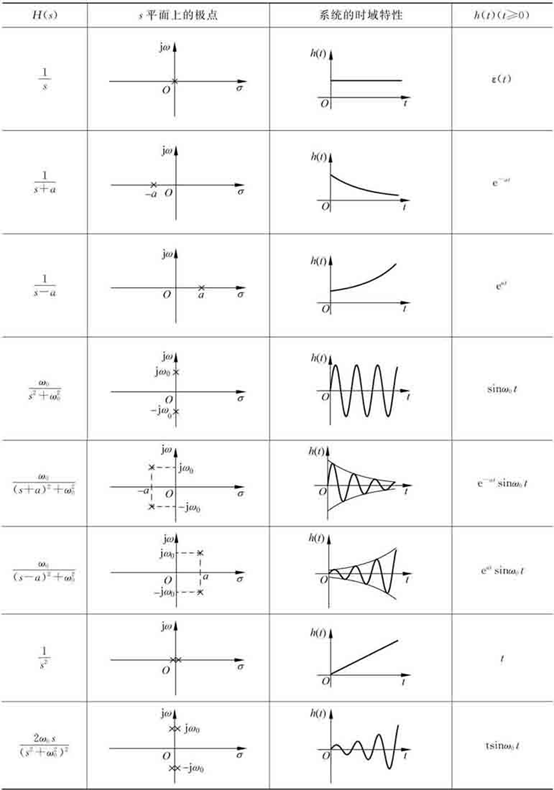%求零极点分布图，冲激响应h(t)和阶跃响应g(t)
%先定义系统函数的分子分母系数向量，然后求零极点，最后用impulse step函数画出波形
num=[1 -2];den=[1 1 0];
sys=tf(num,den);
%%求极点
poles=roots(den)
figure(1)
subplot(231)
pzmap(sys);
%%求响应画图
t=0:0.02:15;
subplot(232);impulse(num,den,t);
subplot(233);step(num,den,t);
%第二个
num=[2 2];den=conv([1 0 1 0],[1 0 1])
sys=tf(num,den);
poles=roots(den)
subplot(234);pzmap(sys);
t=0:0.02:15;
subplot(235);impulse(num,den,t);
subplot(236);step(num,den,t);
%%另一种方法求冲击阶跃响应的表达式并画图
figure(2)
clear
syms t s;
ft=dirac(t)
Fs=laplace(ft)
Bs=s-2;As=s^2+s;
Hs=Bs./As;     %Ys（全响应）=Cs/As(零输入)+Hs*Fs(零状态) 然后用ilaplace求t  冲激响应Fs=1 阶跃响应1/s
ht=ilaplace(Hs*Fs)   %表达式冲激函数
t1=linspace(eps,5,100);
ht1=subs(ht,t,t1);
subplot(211)
plot(t1,ht1);

ft=heaviside(t);
Fs=laplace(ft);
Bs=s-2;As=s^2+s;Hs=Bs./As;
ht=ilaplace(Hs*Fs)   %表达式冲激函数
t1=linspace(eps,5,100);
ht1=subs(ht,t,t1);
subplot(212)
plot(t1,ht1);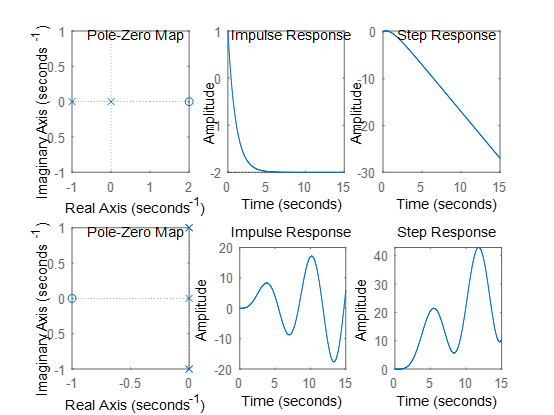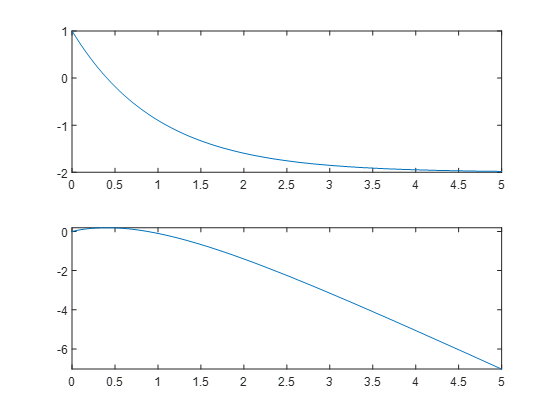零极点分布决定系统的频率特性
%Hs=s-1/(s+1)^2+4 求系统幅频和相频 用freqs函数产生幅频相频特效
num=[1 -1];den=[1 2 5];
sys=tf(num,den);
poles=roots(den)
subplot(2,2,1);pzmap(sys);
axis([-1.5 1.5 -3 3]);
t=0:0.02:10;
h=impulse(num,den,t);
subplot(2,2,2);plot(t,h);
title('Impulse Response')
[H,w]=freqs(num,den);
subplot(2,2,3);plot(w,abs(H))
xlabel('\omega');

title('M agnitude Response')
subplot(2,2,4);plot(w,angle(H))
xlabel('\omega');
title('Phase Response')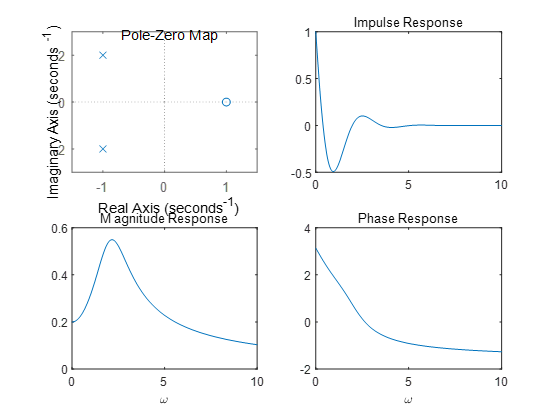系统的稳定性
实际应用中，通常利用H（s）的极点分布来判断系统的稳定性。根据极点的分布状况，系统可分为稳定的、临界稳定的和不稳定的。
（1）稳定系统：若H（s）的极点全部位于s平面的左半平面，则系统是稳定的。
（2）临界稳定系统：若H（s）在原点或虚轴上有单阶极点，其余的极点全部位于s平面的左半平面，则系统是临界稳定的。
（3）不稳定系统：若H（s）的极点不全位于s平面的左半平面，或在原点或虚轴上有高阶重极点，则系统是不稳定的。


展开全文• 精品文档 实验六 拉普拉斯变换及其逆变换 一目的 1掌握连续系统及信号拉普拉斯变换概念 2 掌握利用 MATLAB绘制系统零极点图的方法 3 掌握利用 MATLAB求解拉普拉斯逆变换的方法 二拉普拉斯变换曲面图的绘制 连续时间...
• matlab开发-反拉普拉斯变换变换函数类。该函数可直接用于传递函数的拉普拉斯逆变换。Simulink基础
• 使用两种方法执行拉普拉斯变换的反演：(1) Zakian 方法和 (2) 傅立叶级数近似。 结果与解析解一致。 Zakian 方法提出了超越函数的问题。 在处理振荡函数时，傅立叶级数给出了更好的结果。 在此链接中可以使用 ...
• 该程序为寻找周期函数的拉普拉斯变换而准备。 因此，您需要替换周期的值、周期中的子区间数以及子区间的左端和右端值。
• MATLAB进行拉普拉斯变换
为什么要使用拉普拉斯变换
我们通常把f(t)的拉普拉斯变换写成F(s)，拉普拉斯变换的优点是能把微分方程写成代数方程，而求解代数方程则要简单的多
命令以及步骤

先使用syms 定义变量
使用laplace对函数进行拉普拉斯变换

示例

常数a
syms a
laplace(a)
ans =
1/s^2


幂函数
二次方：
octave:3> syms t;
octave:4> laplace(t^2)
ans = (sym)

2
──
3
s

三次方：
octave:5> laplace(t^3)
ans = (sym)

6
──
4
s

四次方：
octave:6> laplace(t^4)
ans = (sym)

24
──
5
s



公式：指数函数

octave:8> syms t b;
octave:9> laplace(exp(-b*t))
ans = (sym)

1
─────
b + s


正弦函数
octave:10> syms w;
octave:11> laplace(sin(w*t))
ans = (sym)

w
───────
2    2
s  + w


余弦函数
octave:12> syms w;
octave:13> laplace(cos(w*t))
ans = (sym)

s
───────
2    2
s  + w



双曲正弦函数
octave:18> syms w t;
octave:19> laplace(sinh(w*t))
ans = (sym)

w
───────────────
(s - w)⋅(s + w)


双曲余弦函数
 双曲余弦函数需要讨论，比较复杂

octave:22> syms w t;
octave:23> laplace(cosh(w*t))
ans = (sym)

⎧              ⅈ⋅π                  │ 2│
⎪          -s⋅ℯ                     │s │
⎪          ────────             for │──│ < 1
⎪           2    2                  │ 2│
⎪          s  - w                   │w │
⎪
⎪                                   │ 2│
⎪              s                    │w │
⎪           ───────             for │──│ < 1
⎨            2    2                 │ 2│
⎪           s  - w                  │s │
⎪
⎪          ⎛             │  2⎞
⎪  ╭─╮2, 1 ⎜ 1/2    0, 0 │ s ⎟
⎪π⋅│╶┐     ⎜             │ ──⎟
⎪  ╰─╯3, 3 ⎜0, 1/2   0   │  2⎟
⎪          ⎝             │ w ⎠
⎪─────────────────────────────   otherwise
⎩              w


拉普拉斯的变换是线性的
octave:25> f = 5+exp(-2*t);
octave:26> laplace(f)
ans = (sym)

2⋅(3⋅s + 5)
───────────
s⋅(s + 2)




展开全文• 拉普拉斯变换的求逆是求解复杂线性系统的一个非常重要的过程。 函数 f(t)=INVLAP(F(s)) 提供了一种简单、有效和合理准确的方法来获得结果。 它基于论文： J. Valsa 和 L. Brancik：拉普拉斯变换数值反演的近似公式，...
• 来自拉普拉斯变换的 INVLAPFUN 时间函数。 FUN = INVLAPFUN(B,A) 返回一个函数句柄，用于评估与拉普拉斯变换 B(s)/A(s) 关联的时间函数 FUN(t)，其中 B 和 A 是包含多项式系数的相应行向量。 FUN = INVLAPFUN(TF) ...
• matlab拉普拉斯变换与反变换
参考资料
拉普拉斯反变换
命令：ilaplace
>> syms s
>> f=(s-4)/((s-1)*(s-5))

f =

(s - 4)/((s - 1)*(s - 5))
>> ilaplace(f)

ans =

exp(5*t)/4 + (3*exp(t))/4

拉普拉斯变换
命令：laplace
>> syms t
>> f=exp(5*t)/2 + exp(t)/2

f =

exp(5*t)/2 + exp(t)/2

>> laplace(f)

ans =

1/(2*(s - 1)) + 1/(2*(s - 5))

总结
学会syms t定义变量就一切ok。


展开全文• 来自拉普拉斯变换的 INVLAPFUN 时间函数。 FUN = INVLAPFUN(B,A) 返回一个函数句柄，用于评估与拉普拉斯变换 B(s)/A(s) 关联的时间函数 FUN(t)，其中 B 和 A 是包含多项式系数的相应行向量。 FUN = INVLAPFUN(TF) ...
• 三课时精通matlab拉普拉斯变换和逆变换 图像和算法等领域有多年研究和项目...三课时精通matlab拉普拉斯变换和逆变换

图像和算法等领域有多年研究和项目经验；指导发表科技核心期刊经验丰富；多次指导数学建模爱好者参赛。宋星星

¥12.00

立即订阅

扫码下载「CSDN程序员学院APP」，1000+技术好课免费看
APP订阅课程，领取优惠，最少立减5元 ↓↓↓订阅后：请点击此处观看视频课程

视频教程-三课时精通matlab拉普拉斯变换和逆变换-Matlab

学习有效期：永久观看

学习时长：31分钟

学习计划：1天

难度：低

「口碑讲师带队学习，让你的问题不过夜」讲师姓名：宋星星

工程师

讲师介绍：图像和算法等领域有多年研究和项目经验；指导发表科技核心期刊经验丰富；多次指导数学建模爱好者参赛。

☛点击立即跟老师学习☚

「你将学到什么？」

精通matlab拉普拉斯变换和逆变换

「课程学习目录」

1.精通matlab拉普拉斯变换和逆变换前

2.精通matlab拉普拉斯变换和逆变换中

3.精通matlab拉普拉斯变换和逆变换后

「7项超值权益，保障学习质量」

大咖讲解

技术专家系统讲解传授编程思路与实战。

答疑服务

专属社群随时沟通与讲师答疑，扫清学习障碍，自学编程不再难。

课程资料+课件

超实用资料，覆盖核心知识，关键编程技能，方便练习巩固。（部分讲师考虑到版权问题，暂未上传附件，敬请谅解）

常用开发实战

企业常见开发实战案例，带你掌握Python在工作中的不同运用场景。

大牛技术大会视频

2019Python开发者大会视频免费观看，送你一个近距离感受互联网大佬的机会。

APP+PC随时随地学习

满足不同场景，开发编程语言系统学习需求，不受空间、地域限制。

「什么样的技术人适合学习？」

想进入互联网技术行业，但是面对多门编程语言不知如何选择，0基础的你
掌握开发、编程技术单一、冷门，迫切希望能够转型的你
想进入大厂，但是编程经验不够丰富，没有竞争力，程序员找工作难。

「悉心打造精品好课，1天学到大牛3年项目经验」

【完善的技术体系】

技术成长循序渐进，帮助用户轻松掌握

掌握Matlab知识，扎实编码能力

【清晰的课程脉络】

浓缩大牛多年经验，全方位构建出系统化的技术知识脉络，同时注重实战操作。

【仿佛在大厂实习般的课程设计】

课程内容全面提升技术能力，系统学习大厂技术方法论，可复用在日后工作中。

「你可以收获什么？」

学习matlab拉普拉斯变换和逆变换

熟练matlab拉普拉斯变换和逆变换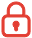订阅课程 开始学习

`
展开全文• 拉普拉斯（laplace）积分变换在工程、应用数学等方面都有重要的作用。用Matlab求解更加方便。 1、拉普拉斯（laplace）变换 语法：F= laplace(f,t,s)%求时域函数f（t）的laplace变换F F是s的函数，参数s省略，返回......

# 拉普拉斯变换matlabmatlab 订阅